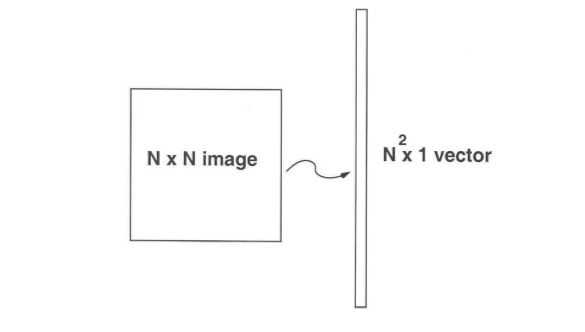# ML | Face Recognition Using Eigenfaces (PCA Algorithm)

In 1991, Turk and Pentland suggested an approach to face recognition that uses dimensionality reduction and linear algebra concepts to recognize faces. This approach is computationally less expensive and easy to implement and thus used in various applications at that time such as handwritten recognition, lip-reading, medical image analysis, etc.
PCA (Principal Component Analysis) is a dimensionality reduction technique that was proposed by Pearson in 1901. It uses Eigenvalues and EigenVectors to reduce dimensionality and project a training sample/data on small feature space. Let’s look at the algorithm in more detail (in a face recognition perspective).

Training Algorithm:

• Let’s Consider a set of m images of dimension N*N (training images).

• We first convert these images into vectors of size N2 such that:• Now we calculate the average of all these face vectors and subtract it from each vector• Now we take all face vectors so that we get a matrix of size of N2 * M.• Now, we find Covariance matrix by multiplying A with AT. A has dimensions N2 * M, thus AT has dimensions M * N2. When we multiplied this gives us matrix of N2 * N2, which gives us N2 eigenvectors of N2 size which is not computationally efficient to calculate. So we calculate our covariance matrix by multiplying AT and A. This gives us M * M matrix which has M (assuming M << N2) eigenvectors of size M.• In this step we calculate eigen values and eigenvectors of above covariance matrix using the formula below.where,andFrom the above statement It can be concluded thatand C have same eigenvalues and their eigenvectors are related by the equation. Thus, the M eigenvalues (and eigenvectors) of covariance matrix gives the M largest eigenvalues(and eigenvectors) of• Now we calculate Eigenvector and Eigenvalues of this reduced covariance matrix and map them into theby using the formula.
• Now we select the K eigenvectors ofcorresponding to the K largest eigenvalues (where K < M). These eigenvectors has size N2.
• In this step we used the eigenvectors that we got in previous step. We take the normalized training faces (face – average face)and represent each face vectors in the linear of combination of the best K eigenvectors (as shown in the diagram below).Theseare called EigenFaces.

• In this step, we take the coefficient of eigenfaces and represent the training faces in the form of a vector of those coefficients.Testing/Detection Algorithm :

• Given an unknown face y, we need to first preprocess the face to make it centered in the image and have the same dimensions as the training face
• Now, we subtract the face from the average face.• Now, we project the normalized vector into eigenspace to obtain the linear combination of eigenfaces.• From the above projection, we generate the vector of the coefficient such that• We take the vector generated in the above step and subtract it from the training image to get the minimum distance between the training vectors and testing vectors

•• If thisis below tolerance level Tr, then it is recognised with l face from training image else the face is not matched from any faces in training set.

• Easy to implement and computationally less expensive.
• No knowledge (such as facial feature) of the image required (except id).

Limitations :

• Proper centered face is required for training/testing.
• The algorithm is sensitive to lightining, shadows and also scale of face in the image .
• Front view of the face is required for this algorithm to work properly.

Reference :

Whether you're preparing for your first job interview or aiming to upskill in this ever-evolving tech landscape, GeeksforGeeks Courses are your key to success. We provide top-quality content at affordable prices, all geared towards accelerating your growth in a time-bound manner. Join the millions we've already empowered, and we're here to do the same for you. Don't miss out - check it out now!

Previous
Next